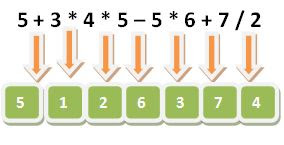### Operators in C Language

Observe the given expression y = x + 5. In the above given expression +, = are the operators and x, y, 5 are called operands / argument.

## Types of operator

According to the required argument the operator can be classified into 3 categories:

1. unary operator: require only one argument. example(-5)
2. binary operator: require two argument. example(5+8)
3. ternary operator: require 3 argument. example(?:)

According to the purpose operator are again classified into 8 types:

1. Arithmetic operator
2. Unary operator
3. Assignment operator
4. Relational operator
5. Logical operator
6. Bitwise operator
7. Conditional operator(ternary operator)
8. Comma operator

### Arithmetic operator

+,-,*,/,%(mod) are called the arithmetic operator.

The +,-,* are same as you learn in mathematical Arithmetic.

To more understand take an example.

5 + 3 * 4

In the above given expression, which operator should be evaluates first? As in mathematics you learn the rule of BODMAS,which tells first brackets should be evaluated, then divide,after that Multiply,addition and subtraction.

In C there are more than 45 operator so BODMAS like rule doesn't not work. For this purpose the Rule of precedence is invented.

### Rule of precedence

In C every operator enjoy the priority, The operator which enjoy the higher priority evaluates first and the operator of lower priority evaluates later.

### Rule of association

The next rule for solving the expression is Rule of association.

This rule is used when in a single expression if there is more than one operator of same priority occurs.

Rule of association tells that some operator are evaluated from left to right, and some other are evaluated from right to left. In the case of left to right associativity, the leftmost operator evaluates first and in the case of the right to left the rightmost operator evaluates first.

### Precedence table of Arithmetic operator

Operator Associativity
* , / , % L to R
+ , - L to R

Example-1:In this example the *(multiply) have the higher priority than (+)addition, so (*)multiply is evaluted before (+)addition.

Example-2:In this example there are multiple operator of same precedence,such as *,/ have the same precedence and +,- have the same precedence.Because *,/ have the higher priority than +,-. so *,/ are evaluated from left to right first and then +,- are evaluated from left to right.